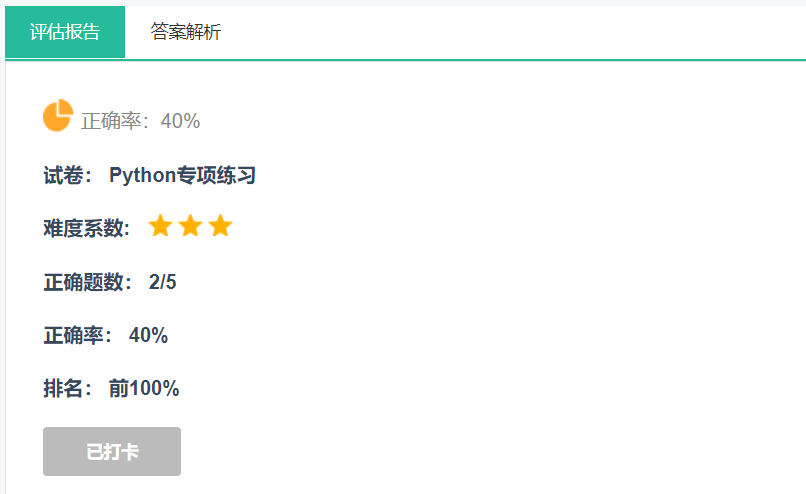# python基础练习（学python的多多少少听说过）

🔝🔝🔝🔝🔝🔝🔝🔝🔝🔝🔝🔝

🥰 博客首页：knighthood2001

😗 欢迎点赞👍评论🗨️

❤️ 热爱python，期待与大家一同进步成长！！❤️

说来惭愧，笔者也自学了很久的python，不过没怎么训练过，都是感兴趣啥就写啥，接下来我会着重刷一下python相关题目。### 判断奇数偶数

``````# Python 判断奇数偶数
# 如果是偶数除于 2 余数为 0
# 如果余数为 1 则为奇数

num = int(input("输入一个数字: "))
if (num % 2) == 0:
print("{0} 是偶数".format(num))
else:
print("{0} 是奇数".format(num))``````

结果如下：

``````输入一个数字: 3
3 是奇数``````

### 判断闰年

``````year = int(input("输入一个年份: "))
if (year % 4) == 0:
if (year % 100) == 0:
if (year % 400) == 0:
print("{0} 是闰年".format(year))   # 整百年能被400整除的是闰年
else:
print("{0} 不是闰年".format(year))
else:
print("{0} 是闰年".format(year))       # 非整百年能被4整除的为闰年
else:
print("{0} 不是闰年".format(year))``````

结果如下：

``````输入一个年份: 2000
2000 是闰年``````
``````输入一个年份: 2011
2011 不是闰年``````

### 质数判断

``````# Python 程序用于检测用户输入的数字是否为质数

# 用户输入数字
num = int(input("请输入一个数字: "))

# 质数大于 1
if num > 1:
# 查看因子
for i in range(2,num):
if (num % i) == 0:
print(num,"不是质数")
print(i,"乘于",num//i,"是",num)
break
else:
print(num,"是质数")

# 如果输入的数字小于或等于 1，不是质数
else:
print(num,"不是质数")``````

结果如下：

``````\$ python3 test.py

1 不是质数
\$ python3 test.py

4 不是质数
2 乘于 2 是 4
\$ python3 test.py

5 是质数``````

### 输出指定范围内的素数

``````# 输出指定范围内的素数
# take input from the user
lower = int(input("输入区间最小值: "))
upper = int(input("输入区间最大值: "))

for num in range(lower,upper + 1):
# 素数大于 1
if num > 1:
for i in range(2,num):
if (num % i) == 0:
break
else:
print(num)``````

### 阶乘

``````# 通过用户输入数字计算阶乘

# 获取用户输入的数字
num = int(input("请输入一个数字: "))
factorial = 1

# 查看数字是负数，0 或 正数
if num < 0:
print("抱歉，负数没有阶乘")
elif num == 0:
print("0 的阶乘为 1")
else:
for i in range(1,num + 1):
factorial = factorial*i
print("%d 的阶乘为 %d" %(num,factorial))``````

结果如下：

``````请输入一个数字: 3
3 的阶乘为 6``````

### 九九乘法表

``````# 九九乘法表
for i in range(1, 10):
for j in range(1, i+1):
print('{}x{}={}\t'.format(j, i, i*j), end='')
print()``````

结果如下：

``````1x1=1
1x2=2    2x2=4
1x3=3    2x3=6    3x3=9
1x4=4    2x4=8    3x4=12    4x4=16
1x5=5    2x5=10    3x5=15    4x5=20    5x5=25
1x6=6    2x6=12    3x6=18    4x6=24    5x6=30    6x6=36
1x7=7    2x7=14    3x7=21    4x7=28    5x7=35    6x7=42    7x7=49
1x8=8    2x8=16    3x8=24    4x8=32    5x8=40    6x8=48    7x8=56    8x8=64
1x9=9    2x9=18    3x9=27    4x9=36    5x9=45    6x9=54    7x9=63    8x9=72    9x9=81``````

### 斐波那契数列

``````# Python 斐波那契数列实现

# 获取用户输入数据
nterms = int(input("你需要几项？"))

# 第一和第二项
n1 = 0
n2 = 1
count = 2

# 判断输入的值是否合法
if nterms <= 0:
print("请输入一个正整数。")
elif nterms == 1:
print("斐波那契数列：")
print(n1)
else:
print("斐波那契数列：")
print(n1,",",n2,end=" , ")
while count < nterms:
nth = n1 + n2
print(nth,end=" , ")
# 更新值
n1 = n2
n2 = nth
count += 1``````

结果如下：

``````你需要几项？ 10

0 , 1 , 1 , 2 , 3 , 5 , 8 , 13 , 21 , 34 ,``````

### 总结

以上的代码及其结果都来自我推荐的那个网址，相信对大多数开始学python的同学来说，应该也都要求写过这些代码，不过该网站上面直接给我们提供了，不需要在额外的去寻找资料了，所以我觉得该网站实在是太棒了。 来试试看吧

后续笔者也会更新这方面的基础知识，敬请期待！！